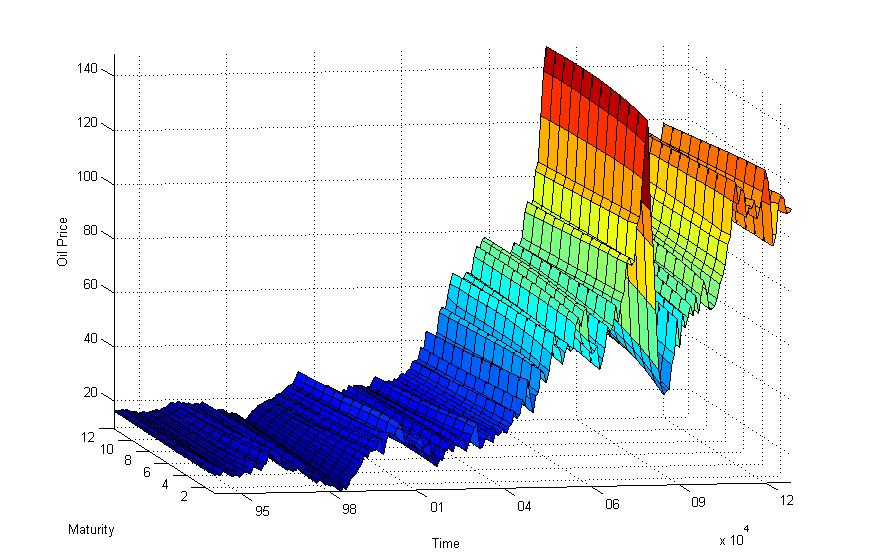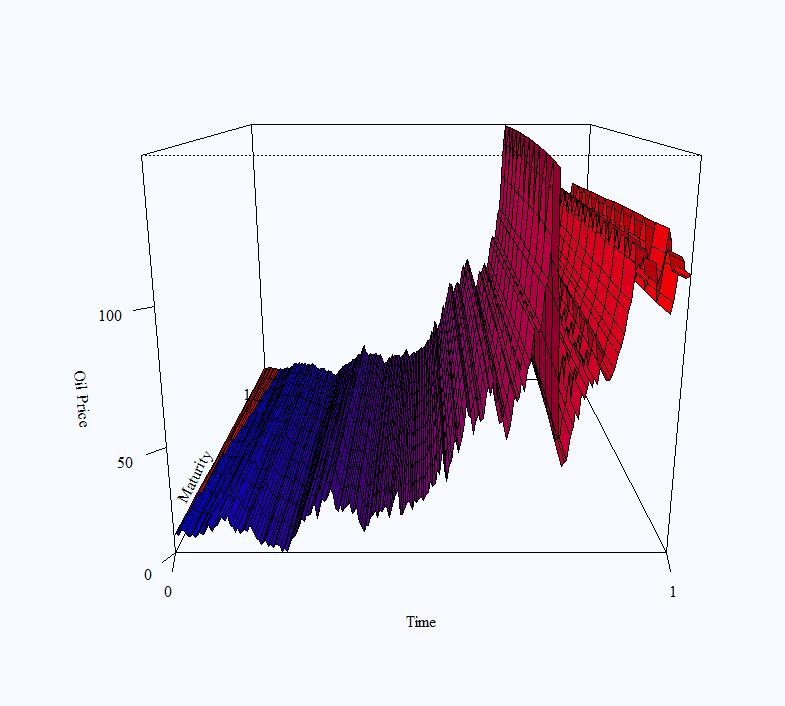# R vs Matlab (round 1)

Matlab has it this time, with solid 3D plotting capabilities.

Here is a figure of the Oil short end (until 12 months) term structure:This was generated very easily via:

R produced less satisfactory result:using the code:

I have also played around with the rgl package which lets you rotate the figure to get a more holistic view, but was straggling to export the figure properly, tips appreciated.

Data for the figures can be found here

### 7 comments on “R vs Matlab (round 1)”

1.gregor says:

Try the Mathnium functions surf and surfl.

Similar to Matlab but free.

2.Ruben Garcia says:

Hi Eran,
thanks for the post.
I’d like to replicate your results but I’m having difficulties loading the data in both: Matlab and R.
May I know what commands are required?
Thanks in advance

1.Eran Raviv says:

In order to replicate you can save the data as a txt file and use read.table to load it into R.
For Matlab, I actually pasted the cells into a variable so no formal command there but you can do the same. In order to create an Excel you can use write.table from R to write the file and then paste it to Matlab.

1.Ruben Garcia says:

Dear Eran,
thanks for the hints. It worked with Matlab but unfortunately with R after using:
dat <-read.table("/Shared/OilCurve.txt")
I got the following error message:
Error in persp.default(dat, col = (colfunc(NROW(dat))), shade = 0.15, :
invalid 'x' argument

Any further suggestions?
Many thanks in advance,
Ruben

2.Eran Raviv says:

### Load Data
dat <- as.matrix(read.table(file = "../OilCurve.txt",header = F)[,2:13] ) ### PLot persp(dat,col=colfunc(NROW(dat)),shade = 0.15, ticktype = "detailed", nticks=2, xlab = "Time", ylab = "Maturity", zlab = "Oil Price",d=1.2,expand = 0.8) ## I hope this works for you, on my end it does.

3.KENDY says:

Dear Eran,
please, can you post the data to replicate the figure in MATLAB?

1.Eran Raviv says:

Dropbox made some changes.. broken link now fixed.